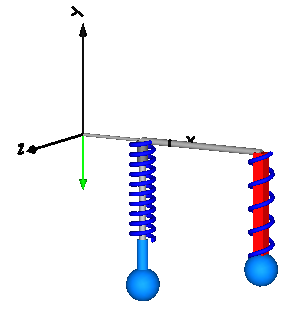# Spring-damper system

Welcome to the spring-damper system example, where we will show you the process of modeling and simulating a basic yet essential mechanical system using the powerful Multibody.jl package in JuliaSim. By understanding the underlying principles of spring-damper systems, you will gain valuable insights into the behavior of various real-world systems, such as suspension systems in vehicles, vibration isolation mechanisms, and biomechanical structures.

This tutorial mirrors that of the following Modelica tutorial Spring damper system and demonstrates that a body can be freely moving without any connection to a joint. In this case body coordinates are used as states by setting the option isroot=true to the body.using Multibody
using ModelingToolkit
using Plots
using SymbolicIR
using OrdinaryDiffEq

t = Multibody.t
D = Differential(t)
world = Multibody.world
@named begin
body1 = Body(; m = 1, isroot = true, r_cm = [0.0, 0, 0], I_11 = 0.1, I_22 = 0.1,
I_33 = 0.1, r_0 = [0.3, -0.2, 0]) # This is root since there is no joint parallel to the spring leading to this body
body2 = Body(; m = 1, isroot = false, r_cm = [0.0, -0.2, 0]) # This is not root since there is a joint parallel to the spring leading to this body
bar1 = FixedTranslation(r = [0.3, 0, 0])
bar2 = FixedTranslation(r = [0.6, 0, 0])
p2 = Prismatic(n = [0, -1, 0], s0 = 0.1, useAxisFlange = true, isroot = true)
spring2 = Multibody.Spring(c = 30, s_unstretched = 0.1)
spring1 = Multibody.Spring(c = 30, s_unstretched = 0.1)
damper1 = Multibody.Damper(d = 2)
end
eqs = [connect(world.frame_b, bar1.frame_a)
connect(bar1.frame_b, bar2.frame_a)
connect(bar2.frame_b, p2.frame_a)
connect(p2.frame_b, body2.frame_a)
connect(bar2.frame_b, spring2.frame_a)
connect(body2.frame_a, spring2.frame_b)
connect(damper1.frame_a, bar1.frame_b)
connect(spring1.frame_a, bar1.frame_b)
connect(damper1.frame_b, body1.frame_a)
connect(spring1.frame_b, body1.frame_a)]

@named model = ODESystem(eqs, t,
systems = [
world,
body1,
body2,
bar1,
bar2,
p2,
spring1,
spring2,
damper1,
])

ssys = structural_simplify(IRSystem(model))

prob = ODEProblem(ssys,
[D.(body1.phi) .=> 0;
D.(D.(body1.phi)) .=> 0;
D(p2.s) => 0;
D(D(p2.s)) => 0;
damper1.d => 2], (0, 10))

sol = solve(prob, Rodas4())
@assert SciMLBase.successful_retcode(sol)

plot(
plot(sol, idxs = [spring1.s, spring2.s]),
plot(sol, idxs = [body1.r_0, body2.r_0]),
plot(sol, idxs = [spring1.f, spring2.f]),
)

This example has two parallel spring-mass parts, the first body (body1) is attached directly to the spring, with no joint in parallel with the spring. In this situation, we have to set isroot=true for body1 to indicate that we want to use the body variables as state. The second body (body2) is attached to the spring with a joint in parallel with the spring, so we can use the joint variables as state, hence isroot=false for body2.